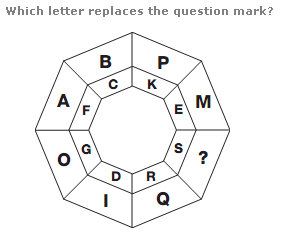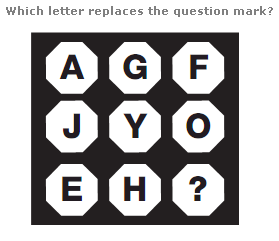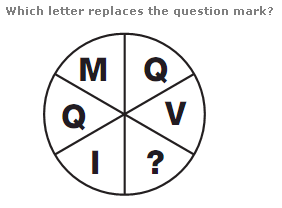# Puzzles - Missing letters puzzles

### Exercise :: Missing letters puzzlesAnswer : N Explanation : Take pairs of letters, one from an outer segment and one from the inner segment in the opposite position. The sum of the numerical values of these letters is always 20.Answer : C Explanation : In each row, the numerical value of the central letter equals the sum of the numerical values of the left and right hand letters.Answer : B Explanation : Starting with the top left segment and moving clockwise, letters advance through the alphabet in steps of 4, then 5, then 6 etc.